• Pytorch List TensorTensor,,reshape拼接等操作.pdf
• 主要介绍了PyTorchTensor拼接与拆分的实现，文中通过示例代码介绍的非常详细，对大家的学习或者工作具有一定的参考学习价值，需要的朋友们下面随着小编来一起学习学习吧
• Pytorch List TensorTensor,reshape拼接等操作 持续更新一些常用的Tensor操作，比如List，Numpy，Tensor之间的转换，Tensor拼接，维度的变换等操作。其它Tensor操作如 einsum等见：待更新。 List TensorTensor...
Pytorch List Tensor转Tensor,reshape拼接等操作
持续更新一些常用的Tensor操作，比如List，Numpy，Tensor之间的转换，Tensor的拼接，维度的变换等操作。其它Tensor操作如 einsum等见：待更新。
List Tensor转Tensor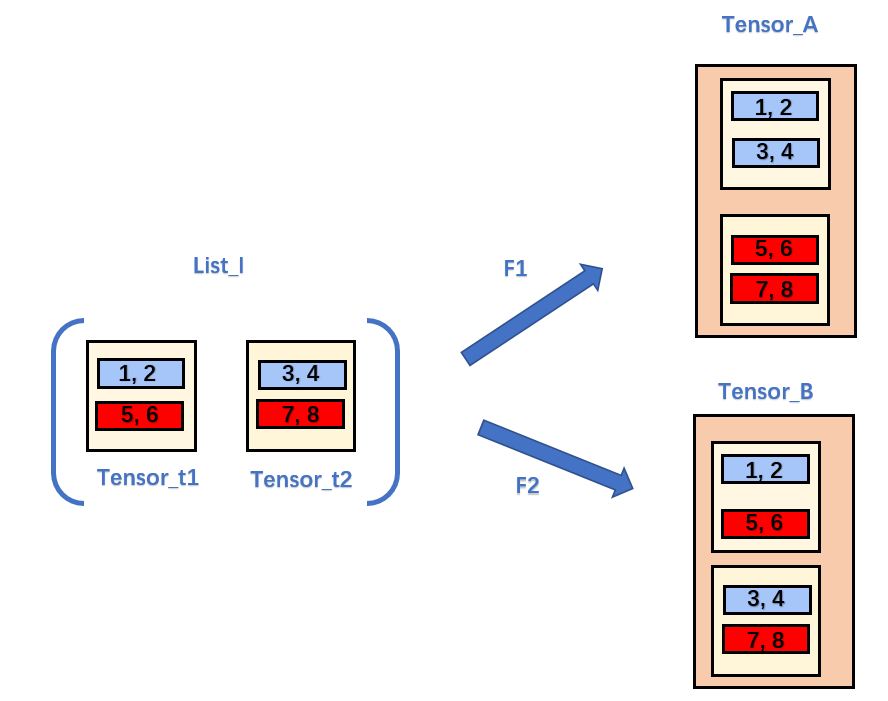// An highlighted block
>>> t1 = torch.FloatTensor([[1,2],[5,6]])
>>> t2 = torch.FloatTensor([[3,4],[7,8]])
>>> l = []
>>> l.append(t1)
>>> l.append(t2)
>>> ta = torch.cat(l,dim=0)
>>> ta = torch.cat(l,dim=0).reshape(2,2,2)
>>> tb = torch.cat(l,dim=1).reshape(2,2,2)
>>> ta
tensor([[[1., 2.],
[5., 6.]],

[[3., 4.],
[7., 8.]]])
>>> tb
tensor([[[1., 2.],
[3., 4.]],

[[5., 6.],
[7., 8.]]])

如果理解了2D to 3DTensor,以此类推，不难理解3D to 4D，看下面代码即可明白：
>>> t1 = torch.range(1,8).reshape(2,2,2)
>>> t2 = torch.range(11,18).reshape(2,2,2)
>>> l = []
>>> l.append(t1)
>>> l.append(t2)
>>> torch.cat(l,dim=2).reshape(2,2,2,2)
tensor([[[[ 1.,  2.],
[11., 12.]],

[[ 3.,  4.],
[13., 14.]]],

[[[ 5.,  6.],
[15., 16.]],

[[ 7.,  8.],
[17., 18.]]]])
>>> torch.cat(l,dim=1).reshape(2,2,2,2)
tensor([[[[ 1.,  2.],
[ 3.,  4.]],

[[11., 12.],
[13., 14.]]],

[[[ 5.,  6.],
[ 7.,  8.]],

[[15., 16.],
[17., 18.]]]])
>>> torch.cat(l,dim=0).reshape(2,2,2,2)
tensor([[[[ 1.,  2.],
[ 3.,  4.]],

[[ 5.,  6.],
[ 7.,  8.]]],

[[[11., 12.],
[13., 14.]],

[[15., 16.],
[17., 18.]]]])

展开全文深度学习
• PyTorchTensor拼接 PyTorchTensor拼接方法：torch.cat() 、torch.stack() 【小提示：代码得到下面的图】 torch.cat() 我们用图+代码来举例 import torch x1 = torch.randn(1, 3) x2 = torch.randn(1, 3) ...
PyTorch中Tensor的拼接
PyTorch中Tensor的拼接方法：torch.cat() 、torch.stack() 【小提示：代码得到下面的图】
torch.cat()
我们用图+代码来举例
import torch
x1 = torch.randn(1, 3)
x2 = torch.randn(1, 3)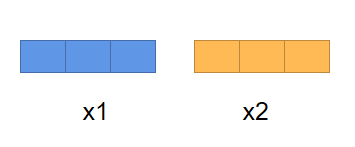# 在 0 维(纵向)进行拼接
torch.cat((x1, x2), 0)
# size
[2, 3]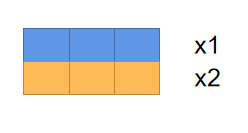# 在 1 维(横向)进行拼接
torch.cat((x1, x2), 1)
# size
[1, 6]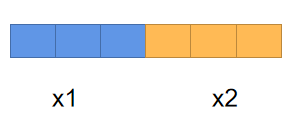注意：对于需要拼接的张量，维度数量必须相同，进行拼接的维度的尺寸可以不同，但是其它维度的尺寸必须相同。【简而言之，堆积木，对上了就可以拼】
torch.stack()
我们继续用图+代码来举例
import torch
x1 = torch.randn(3, 4)
x2 = torch.randn(3, 4)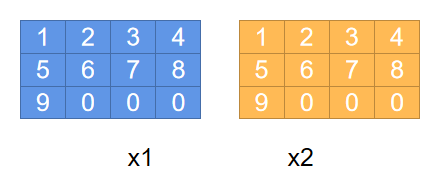# 在 0 维插入一个维度，进行前后组合
torch.stack((x1, x2), 0)
# size
[2, 3，4]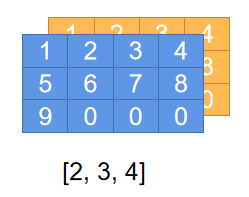# 在 1 维插入一个维度
torch.stack((x1, x2), 1)
# size
[3, 2，4]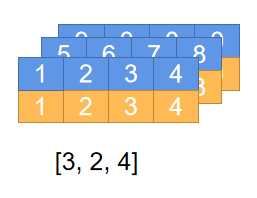# 在 2 维插入一个维度
torch.stack((x1, x2), 2)
# size
[3, 2，4]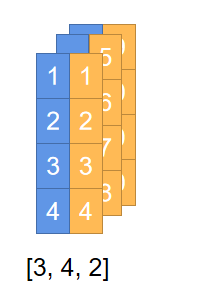补充： 拼接多个向量，例如：torch.stack((x1, x2, x3, x4), 2)，再上述的方法中接入需要拼接的向量就可以了
展开全文python
• Tensor维度的拼接与拆分


文章目录
1. 拼接与拆分常用API2. 按照维度合并Tensor2.1 cat函数2.2 stack函数

3. 按照维度拆分Tensor3.1 split函数3.2 chunk函数

1. 拼接与拆分常用API
cat函数stack函数split函数chunk函数
2. 按照维度合并Tensor
2.1 cat函数
def cat(tensors, dim) -> Tensor

tensors：需要合并的Tensordim：按照维度dim进行合并注意：想要拼接的维度上的值可以不同，但是其它维度上的值必须相同，并且两个 Tensor 的维度最大值必须相同
a = torch.rand(4, 32, 8)  # 含义： 4个班级，每个班级32个人，每个人8门课成绩
b = torch.rand(5, 32, 8)  # 含义： 5个班级，每个班级32个人，每个人8门课成绩

# 合并成绩单
c = torch.cat([a,b], dim=0)
print(c.shape)     #  torch.Size([9, 32, 8])	-> 9个班级，每个班级32个人，每个人8门课成绩


解释：从班级维度( 0D )将成绩进行合并，两个 Tensor 中的 0D 中的值可以不同，但是其他维度上的值必须相同

二维矩阵理解 cat 函数
按行拼接：Tensor1 是 3 行 4 列，Tensor2 是 2 行 4列，将 Tensor1 和 Tensor2 按行拼接，变成 Tensor3 是 5 行 4 列
按列拼接：Tensor1 是 3 行 4 列，Tensor2 是 3 行 5 列，将 Tensor1 和 Tensor2 按行拼接，变成 Tensor3 是 3 行 9 列
2.2 stack函数
def stack(tensors, dim) -> Tensor

tensors：需要合并的Tensordim：将新产生的维度放在 dim 维度注意：需要合并的这些个Tensor，维度个数必须相等，维度中的值也必须相等
a = torch.rand(32, 8)  # 含义： A班级32个人，每个人8门课成绩
b = torch.rand(32, 8)  # 含义： B班级32个人，每个人8门课成绩
c = torch.rand(32, 8)  # 含义： C班级32个人，每个人8门课成绩

# 将三个班级用一个Tensor表示：增加一个维度表示班级
d = torch.stack([a, b, c], dim=0)
print(d.shape)  # torch.Size([3, 32, 8])  -> 含义：3个班级，每个班级32个人，每个人8门课成绩

3. 按照维度拆分Tensor
3.1 split函数
def split(split_size, dim=0)

dim：表示需要拆分的维度split_size
如果是一个数字num，表示将维度为dim中的值按照num进行平均拆分成多个Tensor；如果是一个[num1, num2, num3, …]，表示将该维度中的值按照num进行分配生成指定个数的Tensor 功能：按照某维度的长度来拆分
d = torch.rand(3, 32, 8)  # 含义：3个班级，每个班级32个人，每个人8门课成绩
# 将这个Tensor按照班级维度进行拆分成三个班级Tensor
a, b, c = d.split([1, 1, 1], dim=0)
print(a.shape)  # torch.Size([1, 32, 8]) -> 含义： A班级32个人，每个人8门课成绩
print(b.shape)  # torch.Size([1, 32, 8]) -> 含义： B班级32个人，每个人8门课成绩
print(c.shape)  # torch.Size([1, 32, 8]) -> 含义： C班级32个人，每个人8门课成绩

c = torch.rand(4, 32, 8)  # 含义：4个班级，每个班级32个人，每个人8门课成绩
# 将这个Tensor按照班级维度拆分成两个班级为一个的Tensor
a, b = c.split(2, dim=0)
print(a.shape)  # torch.Size([2, 32, 8]) -> 含义： 该Tensor有2个班级，每个班级32个人，每个人8门课成绩
print(b.shape)  # torch.Size([2, 32, 8]) -> 含义： 该Tensor有2个班级，每个班级32个人，每个人8门课成绩


可能的报错：拆分的值 与 接收Tensor的变量的个数 不合适时
ValueError: not enough values to unpack (expected 3, got 2)
ValueError: too many values to unpack (expected 3)

3.2 chunk函数
def chunk(chunks, dim=0) -> List of Tensors

chunks：要产生Tensor的个数  dim：拆分的维度  功能：将维度为 dim 中的值平均分给chunks个Tensor  按照某维度的数量来拆分
d = torch.rand(6, 32, 8)  # 含义：6个班级，每个班级32个人，每个人8门课成绩
a, b, c = d.chunk(3, dim=0)
print(a.shape)  # torch.Size([2, 32, 8]) -> 含义： 该Tensor有2个班级，每个班级32个人，每个人8门课成绩
print(b.shape)  # torch.Size([2, 32, 8]) -> 含义： 该Tensor有2个班级，每个班级32个人，每个人8门课成绩
print(c.shape)  # torch.Size([2, 32, 8]) -> 含义： 该Tensor有2个班级，每个班级32个人，每个人8门课成绩



解释：将 d 中的 dim 为 0 的维度中的值平均分给 3 个Tensor
故产生的 3 个 Tensor 中 dim 为 0 的值为 6 / 3 = 2

展开全文深度学习
• 修改 第 1 列 数值为 2 # 初始化一个 tensor tensor = torch.ones(4,4) tensor[:,1] = 2 print(tensor) 输出： tensor([[1., 2., 1., 1.], ...横向拼接 t1 = torch.cat([tensor, tensor, tensor],dim = 1) pri.
• Pytorch List TensorTensor,reshape拼接等操作 持续更新一些常用的Tensor操作，比如List，Numpy，Tensor之间的转换，Tensor拼接，维度的变换等操作。其它Tensor操作如 einsum等见：待更新。 List Tensor转...
• 在已有维度上拼接。 import torch a = torch.Tensor([1,2]) b = torch.Tensor([3,4]) c1 = torch.cat((a,b), dim=0) 得到c1为 tensor([1.,2.,3.,4.]) 二.截取 c2 = c1[:,0] 得到c2为 tensor([1.,3.]) 三.拼接 ...
• Pytorch的基础类型——Tensor的简单介绍深度学习...